603 - 5885 1250 (4 lines) info@mes100.com
Select Page

OSD Rational Method (MSMA 1)

1. Project file MiTS 1(PondCAD) here
2. Project file MiTS 2 here
3. Software Report here
5. Linear interpolator here

Objective

1. Comparison between software calculation and manual calculation. To ensure that the results are the same
2. Software extract the correct value from Design Chart/Table in MSMA 1

Project Development details

Area: 0.687 ha

100 m of overland flow

520 m flow in an open drain

Catchment area average slope 1%

MSMA 1st Edition

 OSD Sizing Procedure (MSMA 1 – Rational Method) Manual Excel Spreadsheet MiTS Software 1. Select storage type to be used at the site Below-ground storage Formula used to calculate PSD and SSR (referring to below-ground storage formula) 2. Determine the area of the site that will be drained to the OSD storage system Area: 0.687 ha 100 m of overland flow 520 m flow in an open drain Catchment area average slope 1% MSMA 1st Edition 3. Determine the amount of impervious and pervious areas draining to the OSD storage system Pre-development Impervious = 0 ha   Pervious = 0.687 ha Post-development Impervious = 0.4809 ha Pervious   = 0.2061 ha 4. Determine the time of concentration, tc and tcs Refer design chart 14.1 Nomograph for Estimating Overland Sheet Flow Times, toFrom Design Chart 14.1, tc = to + td = 10 min In MiTS softwareUsing Time of Concentration for Natural Catchment Equation 14.6Calculated from Equation 14.6, tc = 10 min 5(i). Calculate the Pre-Development flows, Qp Pre-Development 1. Calculate rainfall depth,P30 and P60  using Equation 13.2 2. Determine FD from Table 13.3 3. Calculate Rainfall Intensity using Equations 13.3 & 13.4 4. Calculate Qp = CIAP30  = 48.091 mm, P60 = 62.045 mm Pd = 32.32 mm Hence, I = Pd/tc = 32.32 / (10/60) =193.94 mm/hr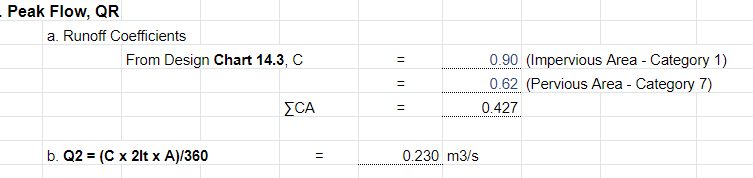Qp = CIA = 0.230 m3/s Pre-Development 1. Calculate rainfall depth,P30 and P60  using Equation 13.2 2. Determine FD from Table 13.3 3. Calculate Rainfall Intensity using Equations 13.3 & 13.4 4. Calculate Qp = CIAP30  = 48.09 mm, P60 = 62.05 mm Pd = 32.32 mm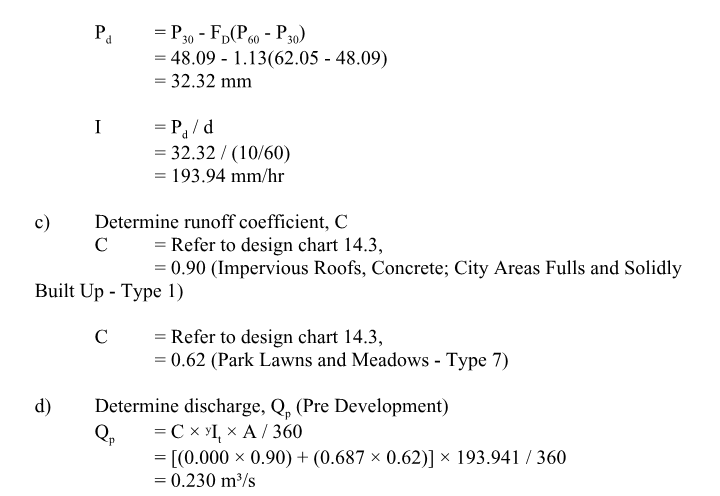Hence, I = Pd/tc = 32.32 / (10/60) =193.94 mm/hr Qp = CIA = 0.230 m3/s 5(ii). Calculate the Post-Development flows, Qa Post-Development1.Calculate rainfall depth,P30 and P60  using Equation 13.2 2. Determine FD from Table 13.3 3. Calculate Rainfall Intensity using Equations 13.3 & 13.4 4. Calculate Qp = CIA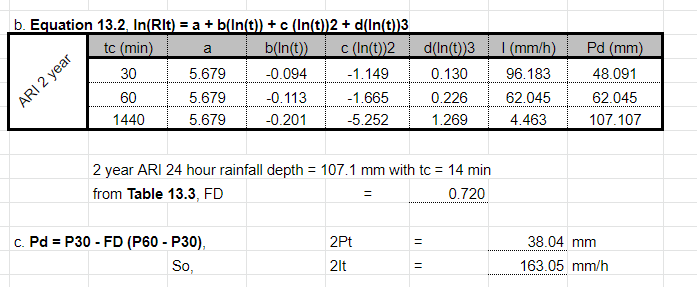P30  = 48.091 mm, P60 = 62.045 mm Pd = 38.04 mm Take tc as nearest = 15 min Hence, I = Pd/tc = 38.04 / (15/60) =152.18 mm/hr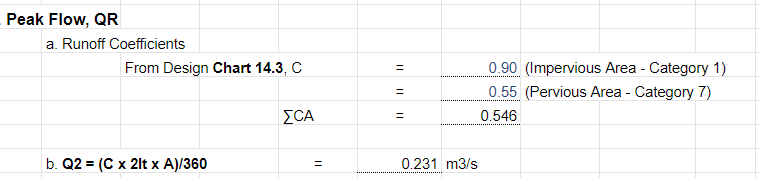Qa = CIA = 0.231 m3/s Post-Development1.Calculate rainfall depth,P30 and P60  using Equation 13.2 2. Determine FD from Table 13.3 3. Calculate Rainfall Intensity using Equations 13.3 & 13.4 4. Calculate Qp = CIA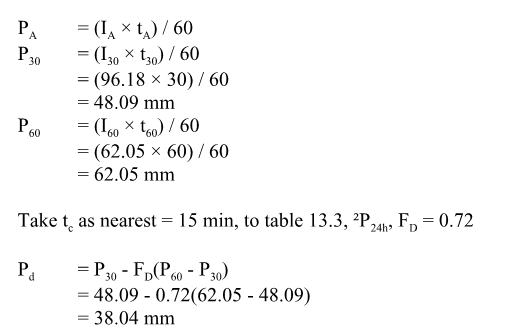P30  = 48.09 mm, P60 = 62.05 mm Pd = 38.04 mmHence, I = Pd/tc = 38.04 / (15/60) =152.18 mm/hr Qa = CIA = 0.230 m3/s 6. Determine the required PSD for the site (Equation 19.1 with Equations 19.1c and 19.1d for below-ground storage)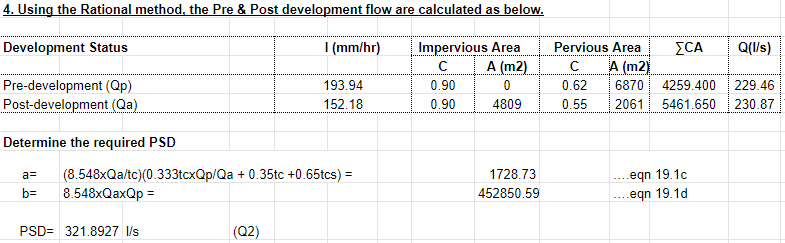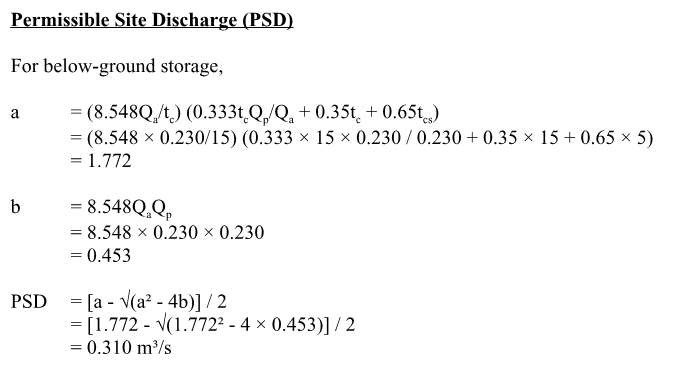7. Determine the required SSR for the site (Equation 19.2 with Equations 19.2c and 19.2d for below-ground storage)Therefore, the required SSR (with 20% added for safety factors) = 157.392 m3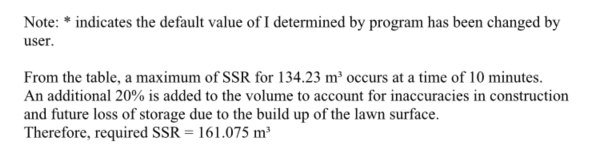Therefore, the required SSR (with 20% added for safety factors) = 161.075 m3 8. Determine storage Dimensions